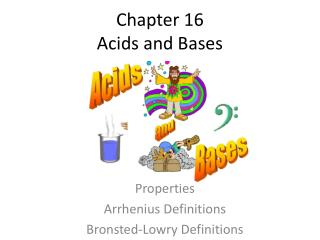DownloadDownload PresentationChapter 16 Acids and Bases

# Chapter 16 Acids and Bases

Télécharger la présentation## Chapter 16 Acids and Bases

- - - - - - - - - - - - - - - - - - - - - - - - - - - E N D - - - - - - - - - - - - - - - - - - - - - - - - - - -
##### Presentation Transcript

1. Chapter 16Acids and Bases Properties Arrhenius Definitions Bronsted-Lowry Definitions

2. Properties of Acids

3. Properties of Bases

4. Svante Arrhenius Acids and Bases • BASES: yield OH- ions in water, substances that increase the OH- ion concentration when dissolved in water • ACIDS: yield H+ ions in water, substances that increase the H+ ion concentration when dissolved in water

5. Bronsted-Lowry Definition • Broadens substances that be categorized as Acid or Base • Involves the TRANSFER of H+ ions

6. Water to Hydronium • Chemists use H+ and H3O+ interchangeably • Considered the “acidic” form of water • Amphoteric = react as an acid or base • Amphiprotic = can donate and accept H+ ion Watch This Tutorial

7. HCl(aq) + H2O (l) Cl-(aq) + H3O+(aq) CONJUGATE ACID BASE ACID CONJUGATE BASE • ACID = proton donor • BASE = proton acceptor

8. Practice Naming/Writing Conjugate Pairs

9. Strengths of Acids and Bases • The stronger an acid, the weaker it’s conjugate base. • INVERSE relationship • Table p. 657 • STRONG acids completely ionize in water, have neglible conjugate bases. • WEAK acids partially ionize in water, have weak base conjugates. • NEGLIBLE acids incapable of protonation (do not dissociate), thus have strong base conjugates.

10. Acid-Base Equilibrium • In every acid-base reaction, equilibrium favors transfer of proton from the stronger acid to the stronger base to form the weaker acid and the weaker base. • In any acid-base reaction, the equilibrium will favor the reaction that moves the proton to the stronger base.

11. HCl(aq) + H2O (l) Cl-(aq) +H3O+(aq) ANSWER: Examine the two bases on table (forward and reverse rxns) H2O and Cl- H2O is a stronger base than Cl-, forward rxn favored, Equilibrium lies to right Kc> 1 QUESTION? Predict whether equilibrium lies to the left or to the right. LEFT would be Kc < 1 RIGHT would be Kc > 1

12. HC2 H3O2(aq) + H2O (l) H3O+ (aq) + C2H3O2- (aq) In any acid-base reaction, the equilibrium will favor the reaction that moves the proton to the stronger base. QUESTION? Predict whether equilibrium lies to the left or to the right. LEFT would be Kc < 1 RIGHT would be Kc > 1 • Acetate is a stronger base than H2O, so the equilibrium favors the left side (K < 1)